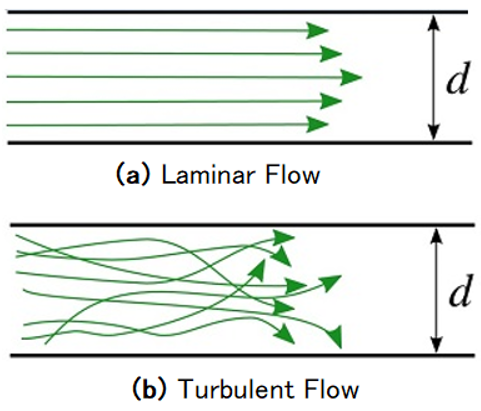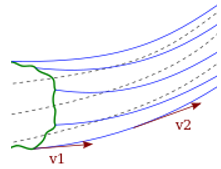# Streamline Flow

• Last Updated : 20 May, 2021

The substance that can change its form under an external force is defined as fluid. Whenever an external force is applied to a fluid, it begins to flow. The study of fluids in motion is defined as fluid dynamics. Have you ever noticed a creek flowing beneath the bridge? When you see a streamline, what is the first thing that comes to mind? The most typical qualities of the stream, such as speed, width, and the volume of water flowing, are the answer. The flow of any fluid is one of the most important properties. The location or source of a stream is a significant factor that determines the flow of water within it. The stream’s source site has the potential to alter both the speed and the direction of flow within the stream.

Another key aspect influencing the stream’s flow is the physical presence of an object in the stream. The object in the stream determines the direction and speed of the flowing water. The velocity of a liquid’s stream flow is its most important feature. As a result of the foregoing qualities, water can be classified as either streamline or laminar flow or turbulent flow.

The fluid can flow in various types; it can be understood by the example of tap water. The water coming from a tap has a smooth appearance while the flow rate is low, but voids and disruptions appear at a certain point when the flow rate is increased.

If one add a stream of ink when the flow is constant, the ink flows without mixing with the other layers, but if one introduce it when the flow is turbulent, the ink layer mix with the other layers of water, as seen in the image below. The first type, referred to as the streamline or laminar flow, will be discussed in this section. And the other is the turbulent flow which will be discussed later.### Streamline Flow

Streamline flow in fluids is described as a flow in which the fluids flow in parallel layers with no interruption or mixing of the layers and the velocity of each fluid particle passing by remains constant over time at a particular position.

There are no turbulent velocity variations at low fluid velocities, and the fluid tends to flow without lateral mixing. The fluid particles move in a specific order with regard to the particles traveling in a straight line parallel to the pipe wall so that the contiguous layers slide past each other like playing cards.

### Streamline

The path traveled by fluid particles under steady flow circumstances is defined as a streamline. The tangent at any point on the curve reveals the direction of the fluid velocity at that moment if the flow lines are represented as curves. The curves, as shown in the figure below, indicate how the fluid particles move in relation to time.

The curve represents the flow of the fluid particles in a steady state. This map is time-stationary, which means that every particle passing a point behaves precisely like the particle that came before it.Representation of Streamline flow

In a laminar flow, the streamlines follow the equation of continuity. i.e.

Av = Constant,

where A is the fluid flow’s cross-sectional area and v is the fluid’s velocity at that location.

The term Av is defined as the fluid’s volume flux or flow rate, which is constant in a steady flow.

Let us consider the cross-sectional area at Point 1 to be A1 and at point 2 to be A2

Then by the equation of continuity:

A1v1 = A2v2

This represents the inverse relationship between area and velocity. The velocity of the liquid decreases as the cross-section area increases, and vice versa.

Moreover, the flow of the water can also be determined by the quantity named Reynold’s number that determines whether a flow pattern is laminar or turbulent which is discussed below:

### Reynolds Number

The Reynolds number is a dimensionless quantity that determines whether a flow pattern is laminar or turbulent as it passes through a pipe. The ratio of inertial forces to viscous forces determines the Reynolds number.

The formula for the Reynolds number is given as:

Re = (p × V × D) / u

where:

• Re is the Reynolds number,
• p is the density of the fluid,
• V is the velocity of flow,
• D is the diameter of the pipe and
• u is the viscosity of the fluid.

Following are the important points for the fluid flow and the Reynolds number:

• The flow through the pipe is said to be turbulent if the computed Reynolds number is high.
• The flow is said to be laminar if the Reynolds number is low.
• These are acceptable numerical values, while laminar and turbulent flows are often grouped within a range.
• Laminar flow has a Reynolds number less than 2000, while turbulent flow has a Reynolds number greater than 2000.

### Sample Problems

Problem 1: On which theorem is the continuity equation based?

Solution:

The flow of the fluid is assumed to be incompressible, laminar and non-viscous for using the continuity equation. The continuity equation is based on the conservation of mass. The product of area and velocity gives the volume flow rate of fluid. Since, the density of fluid is same at all points, the mass of fluid passing through two different regions is same at the same time.

Problem 2: A tube having a uniform cross-section is kept vertical in such a way that water enters from the top and leaves from the bottom. If the speed at a point A below the opening is ‘v’, what will be the speed at a point B vertically below A such that the distance between A & B is ‘2h’?

Solution:

According to the equation of continuity, the velocity at B will be the same as that at A since the area of the cross-section is the same at both points. Although, gravity will increase its velocity, but pressure will also decrease downwards and therefore net effect will be zero change in velocity.

Problem 3: A horizontal pipeline carries water in streamlined flow. At a point along the pipe, where the cross-sectional area is 25 cm^2, the water velocity is 0.5 m/s. Find the velocity of the water at a point where the cross-sectional area is 2 cm^2.

Solution:

Given:

Area at point 1, A1 = 25 cm2

Area at point 2, A2 = 2 cm2

Velocity of point 1, v1 = 0.5 m/s

Let us consider the unknown velocity be v2.

According to continuity equation,

A1v1 = A2v2

Rearrange the formula in terms of v2.

v2 = A1v1 / A2

Substitute all the values in the above expression.

v2 = (25 cm2) × (0.5 m/s) / (2 cm2)

= 6.25 m/s

Thus, the velocity of water at a point of second cross-section is 6.25 m/s.

Problem 4: Determine the flow of fluid having a relative density of 100 kg/m3, the viscosity of 0.5 N s/m2 with a velocity of 5 m/s through a pipe of 0.2 m.

Solution:

The type of flow can be determined by the value of Reynolds number.

Given:

Velocity of fluid, V = 5 m/s

Diameter of pipe, D = 0.2 m

Relative density of fluid, p =100 kg/m3

Viscosity of fluid = 0.5 N s/m2

The formula of Reynolds number is given as:

Re = (p × V × D) / u

Substitute all the values in the formula to calculate the Reynolds number.

Re = (100 kg/m3) × (5 m/s) × (0.2 m) / (0.5 N s/m2)

= 200

Since, the Reynolds number is less than 2000, therefore the flow of liquid is laminar.

Problem 5: Why do two streamlines never intersect?

Solution:

The direction of net velocity of the flow is given by the tangent at a point to the streamline.

If the two streamlines cross, it denotes two different directions of velocity at a point, which are not feasible. As a result, two streamlines cannot intersect.

My Personal Notes arrow_drop_up
Recommended Articles
Page :